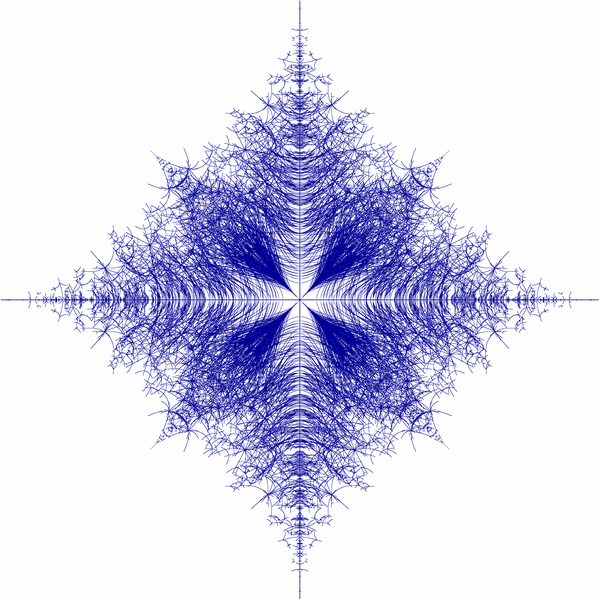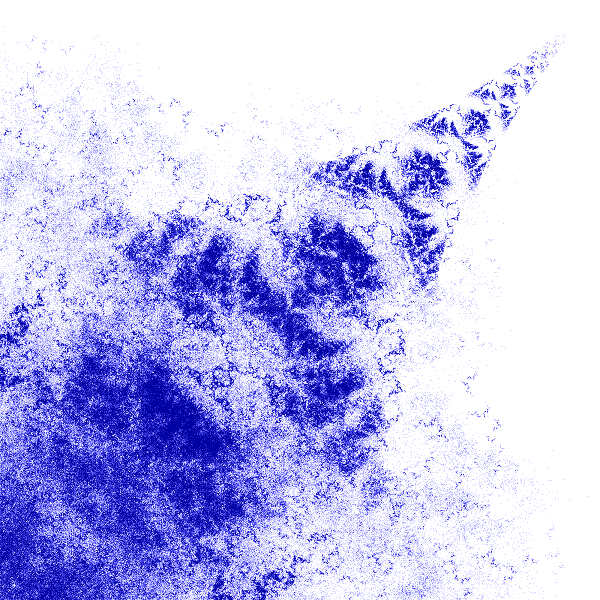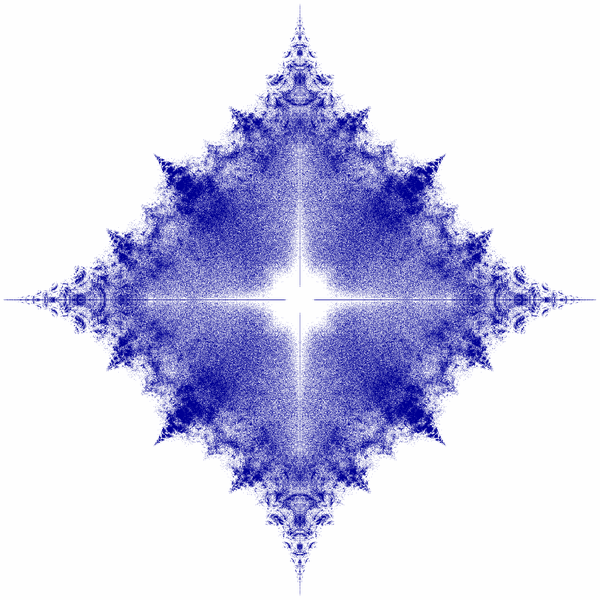# Areas of Research

## Prof. Dr. Marko Lindner

• Fredholm and Spectral theory

We are interested in equations Ax = b, where A is a linear operator acting boundedly between Banach spaces. A is invertible (i.e. it has an inverse, A-1, that is again bounded and linear) if and only if A is injective (its nullspace, the set of solutions x to the homogeneous equation Ax = 0, consists of 0 only) and surjective (its image/range is the whole space). Deviation from both properties, and hence from invertibility, can be measured in terms of the dimension of the nullspace and the codimension of the image. One says that A is a Fredholm operator if both numbers are finite — so injectivity and surjectivity might be violated but only to some (finite dimensional) extent. In particular, Ax = b is solvable for all b in a space of finite codimension and the solution x is unique up to perturbations from a finite-dimensional space.
The Fredholm property is robust under certain perturbations which makes it more accessible than (but still in touch with) invertibility. If you want to prove that your operator A is invertible (i.e. Ax = b has a unique solution x for all b) it is often a good idea (and a big step in the right direction) to start with its Fredholm property.Typical decay properties of infinite matrices under investigation.
left: band matrices, right: norm-limits of such

Our operators A usually appear as infinite matrices (Aij) — possibly with $i,j\in {\mathbf Z}^d$ and Aij operator-valued — usually coming from integral or differential equations (and their discretizations). Our studies on invertibility and Fredholm property give us (in general lower) bounds on spectrum and essential spectrum of A.Spectra of non-selfadjoint Hamiltonian of randomly hopping particle in 1D.

• Numerical Functional Analysis

It is important to know about functional analysis (i.e. linear algebra in infinite dimension) but for most computations one has to resign to finite-dimensional approximations An of our operator A and hence to linear algebra (finite dimensional). These approximations typically come from truncation, interpolation, application of quadrature rules, etc.
We are interested in situations (classes of operators A) for which there is a close connection between properties (spectrum, singular values, pseudospectra, inverse, ...) of the operator A and its finite-dimensional approximations An:

Functional Analysis   vs.    Linear Algebra

Knowing about bridges between these two worlds can be of benefit to both sides: Linear algebra can help to approximately invert A (and hence solve Ax = b), while functional analysis can help to locate the eigenvalues of An (with n = 1023, say) using the spectrum of the operator A.Approximating spec(A) by spectral quantities of An.

In this context we study certain truncation techniques (quadratic, rectangular, shifted, periodized, ...) and their applications in asymptotic inversion and spectral approximation (e.g. upper bounds on spectrum and pseudospectra) of A.Solving acoustic scattering problems in unbounded 3D with modified truncations.

## Dr. Christian Seifert

• Aperiodisch geordnete Modelle

Bei der Untersuchung quantenmechanischer Modelle für Quasikristalle treten seltsame Phänomene auf. Aus dynamischer Sicht gibt es anormalen Transport; aus spektraler Sicht eine Tendenz zu rein singulär stetigen Spektren. Ziel ist es anhand der geometrischen Forderung der aperiodischen Ordnung genau diese spektralen und dynamischen Kuriositäten zu erhalten.
• Quantengraphen

Quantengraphen als Modell für Netzwerkstrukturen bestehen aus einem Graphen, dessen Kanten mit Intervallen identifiziert werden, und einem Differentialoperator, der auf geeignete Funktionen auf den Kanten wirkt. Zusätzlich können Kopplungsbedingungen in den Knoten formuliert werden. Damit lassen sich dann beispielsweise Diffusionen in Netzwerken beschreiben.
• Zufällige Operatoren

Beim Studium ungeordneter oder zufällig gestörter Materialien werden häufig Operatorfamilien genutzt, um viele Realisierungen gleichzeitig beschreiben zu können. Unter geeigneten Homogenitätsbedingungen lassen sich gemeinsame spektrale Eigenschaften der gesamten Operatorfamilie erhalten.